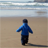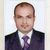New to Qlik Sense

If you’re new to Qlik Sense, start with this Discussion Board and get up-to-speed quickly.

Announcements
QLIKWORLD LIVE! MAY 16 - 19TH, EARLY BIRD DISCOUNTS! REGISTER TODAY
cancel
Showing results for
Did you mean:Creator

Dynamic change of month

Hi

I have data for sales till date months(Jan,Feb,Mar,Apr,May,June,July,Aug).Now I need to compare sales based on current selections of month. For example if I select March month I need to show sum(sales ) for March and previous month Feb sales. Like that current selections of month Vs previous month based on month selections. I needed help on setanalysis.

Thanks,

Raj

1 Solution

Accepted SolutionsMVP

Finally you can select this one

=sum( {<NumMonth ={ \$(=MAX(NumMonth)) }    >}  Sales)

=sum( {<NumMonth ={ \$(=MAX(NumMonth)-1), MonthField= }    >}  Sales)

9 RepliesPartner

Hi Rajesh,

Try

sum({<Month={">=\$(=AddMonths([Month],-1)"}>}Sales) // (In this case, the Month field must be a numerical date, not a string)

This will add for both the previous and current month.

FelipeMVP

Rather than using Getfieldselection() you can use Max for finding maximum month value  in the field same you can apply for less one month but you have to use numeric month field in your data model just add this one line in your data model.

Ex:-

*,

NUM(MonthField) as NumMonth

From Location;

1. Then in expression for Current month what you select

=sum( {<NumMonth ={ \$(=MAX(NumMonth)) }    >}  Sales)

2. For Previous Month use

=sum( {<NumMonth ={ \$(=MAX(NumMonth)-1) }    >}  Sales)

HTH

Regards

AnandMVP

Finally you can select this one

=sum( {<NumMonth ={ \$(=MAX(NumMonth)) }    >}  Sales)

=sum( {<NumMonth ={ \$(=MAX(NumMonth)-1), MonthField= }    >}  Sales)Creator
Author

Thanks it is working fine Anand.. How can we do for week and quarter also..i will open new thread please reply if you have time.

Thanks

RajeshCreator

Try this for week

Sum({<Week = {"\$(=week(max(Date)-7))"}>}Sales)

Sumit Kumar SrivastavaCreator
Author

Thanks..it is working .For quarter Can i do same way..Can you please put expression for Quarter?Partner

Hi Rajesh,

Try this for current Quarter

SUM({<DATE={">=\$(=QuarterStart(Max(DATE)))<=\$(=QuarterEnd(date(Max(DATE))))"}>} sales)Creator
Author

Hi Sumit ,

I  needed quarter current selection and previous selection like below format.it helps me alot

sum( {<NumMonth ={ \$(=MAX(NumMonth)) }    >}  Sales)

=sum( {<NumMonth ={ \$(=MAX(NumMonth)-1), MonthField= }    >}  Sales)

Thanks,

RajCreator

Sum({<QuarterYear=,Year=,Date = {">=\$(=Quarterstart(max(Date), -5))<=\$(=QuarterEnd(Max(Date)))"}>} Sales)

Sumit Kumar SrivastavaTags
Community Browser